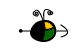Using Integrated Rate Laws

We use integrated rate laws, and rate constants to relate concentrations and time. The rate law to use depends on the overall order of the reaction.

The Common Integrated Rate Laws

For a zero order reaction:     Aproducts   ,    rate =  k

The integrated rate law is   [A] = -kt + [Ao]
For a first order reaction:      Aproducts  ,   rate =  k[A]
The integrated rate law is   ln [A] = -kt + ln [Ao]
For a second order reaction:   2Aproducts   or   A + Bproducts   (when [A] = [B])    ,    rate = k[A]2
The integrated rate law is  1/[A] = kt + 1/[Ao]

Top

How Long Does it Take?

To determine t, the time required for the initial concentration of a reactant to be reduced to some final value, we need to know:

• The initial concentration, [Ao].
• The final concentration, [A].
• The order of the reaction or enough information to determine it.
• The rate constant, k, for the reaction or enough information to determine it.

Substitute this information into the integrated rate law for a reaction with this order and solve for t. The integrated rate laws are given above.

Top

How Much Remains After a Given Time?

To determine [A], the concentration of a reactant remaining after some time, t, we need to know:

• The initial concentration, [Ao].
• The length of time the reaction ran, t.
• The order of the reaction or enough information to determine it.
• The rate constant, k, for the reaction or enough information to determine it.

Substitute this information into the integrated rate law for a reaction with this order and solve for [A]. The integrated rate laws are given above.

Top

What Concentration Was Present Initially?

To determine [Ao], the initial concentration of a reactant, we need to know:

• The final concentration, [A].
• The length of time, t, the reaction ran to reach the final concentration.
• The order of the reaction or enough information to determine it.
• The rate constant, k, for the reaction or enough information to determine it.

Substitute this information into the integrated rate law for a reaction with this order and solve the equation for [Ao]. The integrated rate laws are given above.

Top